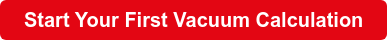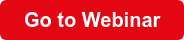# Calculating Evacuation Time for Vacuum Chambers

By LeyboldOne of the most common applications of vacuum pumps is the evacuation of vacuum chambers. This process removes gas from closed tanks in a given time by lowering the pressure in a chamber from a starting pressure to a target pressure.

Calculating vacuum chamber evacuation time with accuracy is a challenging task, no matter who you are. Here are the basics you need to know:

Calculating Vacuum Chamber Evacuation Time

We can use the simple logarithmic equation below to calculate estimated evacuation times where V is the vessel's volume, S is the pumping speed, and p1 and p2 represent the start and target pressure respectively:

t = (V / S) * ln(p1 / p2)

However, this simplified approach doesn’t consider various factors:

• Pumping speed
• Conductance
• Thermal effects
• Desorption

Each of these factors impacts evacuation time.

Pumping Speed

The simplified equation assumes a constant suction speed. We can perform a more accurate calculation by applying this equation to multiple segments of the typical speed curve of a specific pump type.

Each of these potential pump types will have a unique impact on evacuation time:

• Oil-lubricated rotary vanes
• Dry scrolls
• Screws
• Multistage roots

Conductance

Conductance measures the degree to which an object conducts electricity, calculated as the ratio of flowing current to the potential difference. It’s the reciprocal of resistance and is measured in siemens or mhos

We must consider the conductance of pipe works, valves, and other system components when calculating vacuum chamber evacuation time. Each connected component between a pump and a vacuum vessel reduces effective pumping speed and increases evacuation time.

Bear in mind that vacuum pipes add volume to the system and must also be evacuated.

Thermal Effects

The internal energy and temperature of the remaining gas are impacted when gas mass is removed from a volume. In slow evacuation processes, the temperature drop is compensated by a heat transfer from the chamber walls.

However, if the process is faster than a few minutes, the gas that has to be pumped will gradually cool. This cooling will reduce the effectiveness of the system's pumping speed.

Desorption

Desorption is the release of an adsorbed substance from a surface. All materials in a vacuum release gases from their surfaces. Water vapor is the primary component of desorption, but lubricants can also impact desorption rates.

Desorption is the dominant source of gas in high and ultra-high vacuum conditions.

Tip: Use our online calculator to simulate systems of pumps, components and chambers. It can take desorption rates into account when calculating evacuation time. Click the button below and use LEYCALC.To calculate the evacuation time for a vacuum chamber, all of the above factors must be taken into account.

For a quick estimate, however, it is often sufficient to perform a segmented use of the simplified evacuation time equation.

This formula gives you the ideal evacuation time, but it will likely be longer depending on the specific contributing factors. It’s also important to note that these results can only be regarded as a reasonable estimate for rough vacuums. Fine vacuum pressure ranges from 1000mbar to approx. 0.1mbar.

### Summary

When calculating vacuum chamber evacuation times, you can perform a basic calculation to get a rough idea. You must consider pumping speed, conductance, thermal effects, and desorption when you need a more accurate measurement.

For a more accurate vacuum system calculation, we recommend using the Leybold calculator "LEYCALC". To find out more, click the button below and watch our free on-demand webinar, on LEYCALC An Online Tool to Calculate Vacuum Performance.Tags: R&D

### Related Articles

Topics: R&D